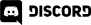# Home > Programming >

31. October 2014

Now that we know how to create vector graphics on iPad, let's take a look at the process of getting them into and using them in Codea.  This part is insanely easy, in fact if this was all there was to it, I could cover this entire process in a single Tweet!  Let’s do it.

First of course you need to create a vector graphic in some application and save it as PDF to your Dropbox folder.

Then fire up Codea, create a new project with the following code:

```function draw()
background(40, 40, 50)
sprite("Dropbox:Jet",WIDTH/2,HEIGHT/2)
end
```

Yeah... that's it.  Select the image from your Dropbox, run it and you will see:So basically vectors are treated exactly the same as bitmaps as far as Codea is concern.  Well, with a few exceptions… you can scale them as much as you want and never lose visual fidelity.

You can scale by passing in the width and height to the sprite call, like so:

```sprite("Dropbox:Jet",WIDTH/2,HEIGHT/2,100,100)
```

So then, vectors are easy to load, look great, scale well are pretty easy to draw... perfect no?

Well… no, there is a catch.  Performance.

Let’s take a quick look at how vectors perform, using the following code:

```-- VectorTest
function setup()
fontSize(160)
fill(255, 0, 25, 255)
end

function draw()
background(40, 40, 50)

local outString = "fps:" .. math.floor( 1/DeltaTime)
local dimensions = textSize(outString)

strokeWidth(5)

for i= 1,100 do
local x = math.random(WIDTH)
local y = math.random(HEIGHT)
sprite("Dropbox:Jet 2",x,y)
end

text(outString,WIDTH-dimensions/2,80)
end
```

Here it is running on my iPad Air with drawing 100 vectors:60 FPS is as good as we can get, so so far so good!  Now lets see what happens when we crank the number up to 500.Oh…

From my tests, you can draw about 200 vector images before framerate starts to drop down.  You can easily draw twice that many normal sprites without seeing a noticeable drop in framerate.

Right now our simple frame rate counter is changing far too often to be of much use.  So instead I am going to sample and average across minutes.  Basically every frame we take a sampling of framerate, after a second elapses we move that sample to other array, average and display the results.  So basically we sample the data per frame, but average it out over the span of a second.  This should give us a much smoother frame rate total.  Here is the updated code:

```-- VectorTest
frameRateSamples = { 60 }
fpsSampledPerSecond = { 60 }
elapsedTime = 0

function setup()
fontSize(160)
fill(255, 0, 25, 255)
end

function mean( t )
local sum = 0
local count= 0

for k,v in pairs(t) do
if type(v) == 'number' then
sum = sum + v
count = count + 1
end
end

return math.floor(sum / count)
end

function draw()
background(40, 40, 50)

elapsedTime = elapsedTime + DeltaTime
if(elapsedTime < 1) then
table.insert(frameRateSamples,math.floor(1/DeltaTime))
else
print("Second elapsed")
table.insert(fpsSampledPerSecond,mean(frameRateSamples))
framerateSamples = {}
elapsedTime = 0
-- ever 360 seconds reset the table so it doesnt grow forever
if #fpsSampledPerSecond > 360 then
fpsSampledPerSecond = { 60 }
end
end

local outString = "fps:" .. mean(fpsSampledPerSecond)
local dimensions = textSize(outString)

strokeWidth(5)

for i= 1,200 do
local x = math.random(WIDTH)
local y = math.random(HEIGHT)
sprite(“YourImageHere",x,y)
end

text(outString,WIDTH-dimensions/2,80)
end

```

Now when we run it, we get the follow values, after letting each run for over 60 seconds to take the seed value out of the equation:

 Image Type FPS at 100 FPS at 250 FPS at 500 FPS at 1000 FPS at 1500 FPS at 2000 Vector 58 38 21 23 15 9 Bitmap 58 37 21 21 17 10

Hmmm, that's interesting.  So once the framerate sampling is smoothed out, the performance between vector and bitmap graphics is basically identical.  In both cases too, oddly enough, 500 sprites caused a drop in performance compared to 1,000…  very very very odd.

Now let’s take a look at if scaling is a factor.  In this test we will scale the vector down to 32x32, vs drawing a fixed 32x32 sprite and see if it impacts performance.

As a 32x32 pixel image, at 2000 sprites, the framerate a solid 57.

As a vector scaled down to 32x32 in the sprite call the framerate at 2000 sprites is 57.

There you have it, contrary to my initial findings, the performance between identically sized bitmap and vector images in Codea is about the same.  Internally, Codea must be rasterizing vectors before drawing them.

So, why did my initial tests show a massive improvement in bitmap performance over vectors as the count increased?  Well, that’s because I compared apples to oranges… I used a “nearly the same dimensions” graphic for the test, and that was a huge mistake. Something critical you can take away from this experiment is the size of the sprite being blitted has a huge impact on performance, much more so than the type of sprite.  As you saw above, we can blit > 2000 32x32 pixel images without a drop in performance, while the same number of 200x200 pixel images brings it to it’s knees.

So, in a nutshell, its equally as valid to use a vector with code than it is a bitmap.  And as you can see, it uses the exact same code.  Oh, and if you are by chance wondering why we were toping out at 57-58FPS instead of 60 you would expect, that is simply because I floored() the results (aka, rounded down) to make the numbers prettier.  Since I was measuring relative performance, not overall performance, the actual framerate really didn’t matter all that much.